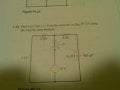# Find Vc(t) using first order circuits

#### blalaw

Joined Oct 28, 2013
9
I am doing a homework problem to solve for the voltage across the capacitor. Our teacher gave us a seven step method to solve for them and told us not to worry about the differential equation way right now.We have to find the Vc(t) when t < 0 and when t = ∞. I can find Vc(t) when t = ∞ easy enough but when I try to find when t < 0 I am not getting the right answer according to the book.

I try to simplify the circuit down and get a resistance of 4K to the left and then a single resistor to the right of 2k. Once I have that I use voltage division to get the voltage across Vc(t) = 4V but the book is saying its 12V and i am just not sure why or where I am going wrong. Any help or explanation would be appreciated.

#### WBahn

Joined Mar 31, 2012
26,466
Why are you using voltage division? Do you have a voltage divider? Go back and look at what a voltage divider is.

Just because you have a circuit that has a voltage source and two resistors in it and just because you have an equation that uses a voltage and two resistances, doesn't mean that you can throw those numbers into that equation and get anything that means anything out of it.

#### t_n_k

Joined Mar 6, 2009
5,455
Suppose the capacitor voltage was 4V at t=∞. This would notionally be the steady state final value. The voltage at the top of the closed switch would be 12V as fixed by the 12V source. So the 2kΩ in series with the capacitor would have 12V on one side (from the 12V source through the closed switch) and 4V (from the capacitor) on the other side. So there would be current in the 2kΩ resistor flowing down into the capacitor. So the capacitor voltage would be increasing. Which is a nonsense since we have already stated the capacitor voltage is at a steady state value of (albeit incorrect) 4V. So the final capacitor voltage can't be 4V.

It should hopefully be apparent that the two 2kΩ resistors in series in the left hand side branch play no part in the charging of the capacitor. They simply ensure that (given sufficient time before switch closure) any initial charge on the capacitor would have been discharged prior to the closure of the switch.

Last edited:

#### blalaw

Joined Oct 28, 2013
9
I believe I do understand why Vc(t) = 12V now. Since the capacitor is an open circuit when doing the analysis there is no current through the right side loop so therefore there is no voltage associated with the resistor so we act like it inst even there. So once I assume that then Vc(t) is equal to the 12V. Is that correct?

#### WBahn

Joined Mar 31, 2012
26,466
I believe I do understand why Vc(t) = 12V now. Since the capacitor is an open circuit when doing the analysis there is no current through the right side loop so therefore there is no voltage associated with the resistor so we act like it inst even there. So once I assume that then Vc(t) is equal to the 12V. Is that correct?
That's correct.

#### Dr.killjoy

Joined Apr 28, 2013
1,196
That's correct.
I just learned that when reading my electronics book ...

Make: Electronics (Learning by Discovery)#### donpetru

Joined Nov 14, 2008
185
Vc(t) is the voltage on the capacitor, which at time t > 0 will be:

Vc(t) =U * (1 - e^(-t/(R*C))); where U= 12Vdc; R = 2 kOhm, C = 100uF;

If the switch opens, the voltage on the capacitor is given by:

Vc(t) =U * e^(-t/(R*C)); where U= 12Vdc; R = 6 kOhm, C = 100uF.

#### WBahn

Joined Mar 31, 2012
26,466
Vc(t) is the voltage on the capacitor, which at time t > 0 will be:

Vc(t) =U * (1 - e^(-t/(R*C))); where U= 12Vdc; R = 2 kOhm, C = 100uF;

If the switch opens, the voltage on the capacitor is given by:

Vc(t) =U * e^(-t/(R*C)); where U= 12Vdc; R = 6 kOhm, C = 100uF.
It's bad enough when people ask someone to do their homework for them and someone obliges and does so. In this case, the OP asked for help understanding a very specific part of getting started on the problem and got that help and has indicated that, at least for now, they are in good shape. But still someone comes in and gives them the answer to whole thing. Perhaps you could arrange to take their exams for them, too.

#### donpetru

Joined Nov 14, 2008
185
Perhaps you could arrange to take their exams for them, too.
No, I could not do that but you better leave this cynicism.
On the other hand, I understand that you want to tell me it's not fair to formulate the exact solution of the problem, on the contrary, it's good to give the user a bibliography needs, such as: